Triangles in Circles

The following pictures show n equilateral triangles with side 1 packed inside the smallest known circle (of radius r).

 1.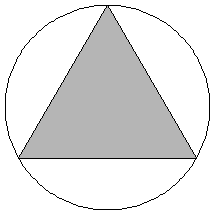2.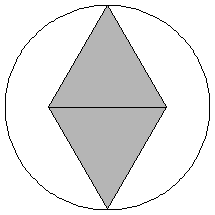3.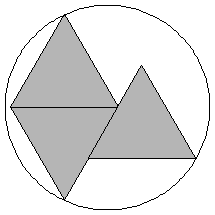r = 1/√3 = .577+Trivial. r = √3/2 = .866+Trivial. r = 3√3 - 3√2 = .953+Found by Erich Friedmanin 1997.

 4.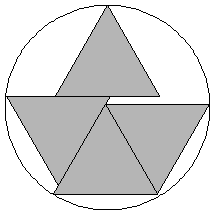5.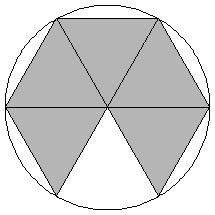6.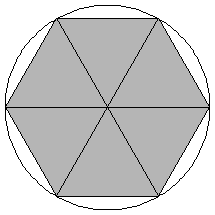r = .980+Found by Erich Friedmanin 1997. r = 1Trivial. r = 1Trivial.

 7.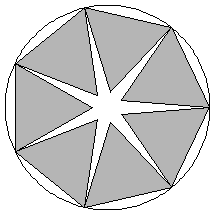8.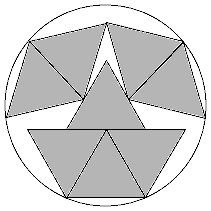9.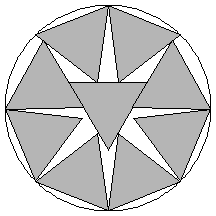r = 1.152+Found by Erich Friedmanin 1997. r = 1.263+Found by Maurizio Morandiin April 2008. r = 1.314+Found by David W. Cantrellin July 2002.

 10.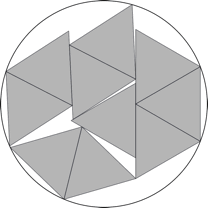11.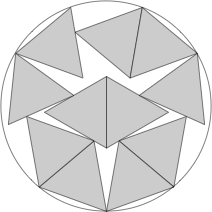12.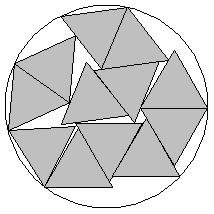r = 1.385+Found by David W. Cantrellin July 2002. r = 1.466+Found by DC after MMin August 2012. r = 1.507+Found by David W. Cantrellin October 2005.

 13.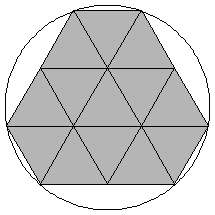14.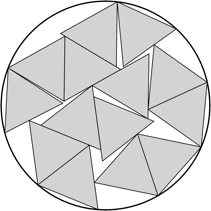15.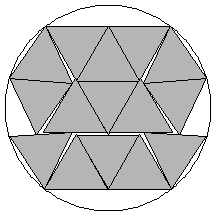r = √(7/3) = 1.527+Found by Erich Friedmanin 1997. r = 1.609+Found by DC after MMin August 2012. r = 1.636+Found by David W. Cantrellin July 2002.

 16.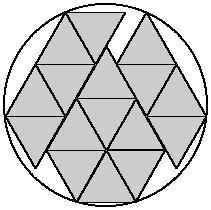17.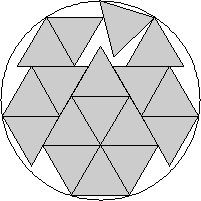18.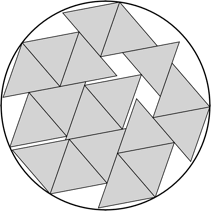r = 1.687+Found by Maurizio Morandiin April 2008. r = 1.730+Found by David W. Cantrellin July 2005. r = √13/2 = 1.802+Found by Erich Friedmanin 1997.

 19.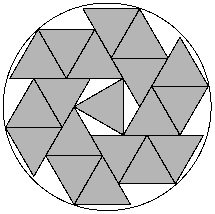20.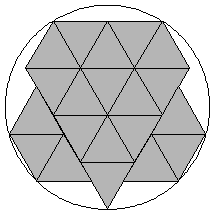21.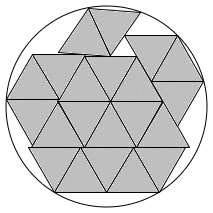r = √(10/3) = 1.825+Found by Haym Hirshin August 2019. r = 1.874+Found by Erich Friedmanin 1997. r = 1.910+Found by Maurizio Morandiin May 2008.

 22.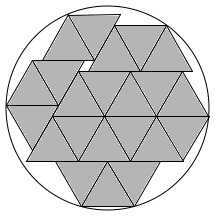23.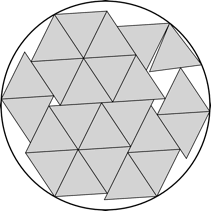24.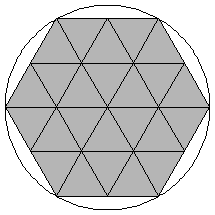r = 1.943+Found by Maurizio Morandiin June 2008. r = 1.988+Found by Maurizio Morandiin June 2008. r = 2Trivial.

 25.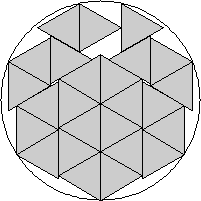26.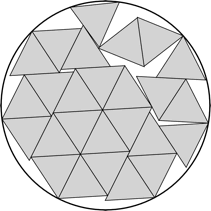27.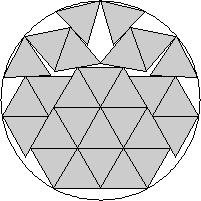r = 2.055+Found by David W. Cantrellin July 2005. r = 2.109+Found by Maurizio Morandiin April 2008. r = 2.138+Found by David W. Cantrellin July 2005.

 28.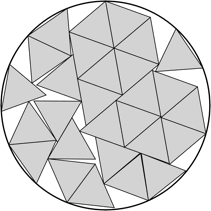29.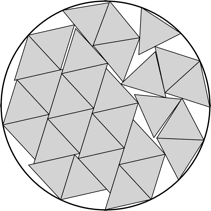30.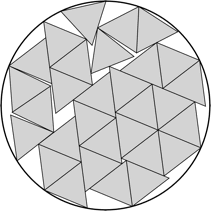r = 2.191+Found by David W. Cantrellin July 2005. r = 2.215+Found by Maurizio Morandiin June 2008. r = 2.258+Found by Maurizio Morandiin July 2008.

 31.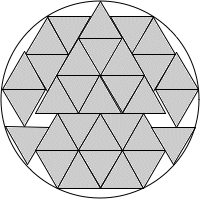r = 2.272+Found by Maurizio Morandiin July 2008.